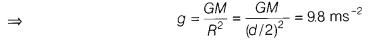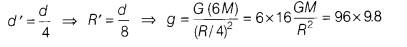# How much the weight of the object on surface of the Earth be affected?

Consider a case in which the diameter of the Earth becomes one-fourth of its present value and the mass becomes six times its present value, then how much the weight of the object on surface of the Earth be affected?

Weight, w = mass (m) x acceleration due to gravity (g)
When mass is constant, w ∝ gBut when, M’ = 6M
andThis means, w becomes 96 times of g.